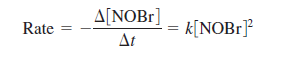Chemistry Practice Problems Identifying Reaction Order Practice Problems Solution: The rate law for the reaction 2NOBr (g) → 2NO (g) ...

⚠️Our tutors found the solution shown to be helpful for the problem you're searching for. We don't have the exact solution yet.

# Solution: The rate law for the reaction 2NOBr (g) → 2NO (g) + Br2 (g)at some temperature isa. If the half-life for this reaction is 2.00 s when [NOBr] 0 = 0.900 M, calculate the value of k for this reaction.

###### Problem

The rate law for the reaction

2NOBr (g) → 2NO (g) + Br2 (g)

at some temperature isa. If the half-life for this reaction is 2.00 s when [NOBr] 0 = 0.900 M, calculate the value of k for this reaction.

Identifying Reaction Order

Identifying Reaction Order

#### Q. At 700 K acetaldehyde decomposes in the gas phase to methane and carbon monoxide. The reaction is: CH3CHO(g) → CH4(g) + CO(g). A sample of CH3CHO is h...

Solved • Thu Dec 06 2018 09:35:18 GMT-0500 (EST)

Identifying Reaction Order

#### Q. Indicate the order of reaction for each of the following observations.A plot of the concentration of the reactant versus time yields a straight line.

Solved • Thu Dec 06 2018 09:35:14 GMT-0500 (EST)

Identifying Reaction Order

#### Q. A certain reaction has the following general form: aA → bB At a particular temperature and [A]0 = 2.00 x 10-2 M, concentration versus time data were c...

Solved • Wed Nov 07 2018 16:03:12 GMT-0500 (EST)

Identifying Reaction Order

#### Q. For the reaction A → products, successive half-lives are observed to be 10.0, 20.0, and 40.0 min for an experiment in which [A]0 = 0.10 M. Calculate t...

Solved • Mon Nov 05 2018 18:38:03 GMT-0500 (EST)